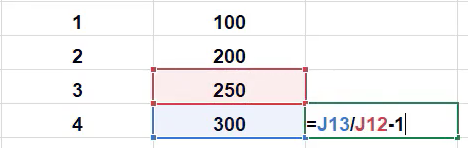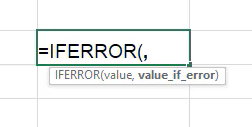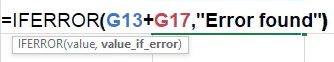# Formula Errors

In this chapter, we’re going to take a look at some of the common errors that occur when formulas are entered into Excel incorrectly.

Use this workbook for the chapter.

Note: This is the same workbook used in the If Statements chapter.

## Four Common Errors

A common problem that can produce errors is misspelling. For instance, let’s say we’d like to take the average of all of the ages. If you complete the formula but “average” is spelled incorrectly, Excel cannot recognize the formula itself. It will give you an error that says “#NAME?” This can be corrected by typing in the correct spelling of the formula and hitting Enter.

Another type of error is called the value error. Let’s say you are trying to calculate the sum of a few random cells, but rather than using the sum formula, you just select cells and put the plus sign between them. If you select a cell that is text rather than numbers, it will show you an error that says, “#VALUE!” This is because it cannot calculate text. However, if you use the =SUM formula and include a text cell, it will calculate the sum because it has the capability to add together only the numeral cells. In the case of a value error, simply get rid of the cell that contains text.

A third error type is the divide-by-zero error. For instance, if you type, “=100/0,” it will give you an error that says, “#DIV/0!” Since Excel can’t calculate an infinite number, it gives this error. For example, this could occur with sales history. If you’re trying to calculate the difference in sales between four years, in year zero, you have no “last-year’s sales.” For example, in the following data, if you divide the new year by the old year and Subtract one, it will give you the percentage increase. (See Figure 18.1)Figure 18.1

However, if you drag that formula up to the other years, when it gets to 1, there is no former year to divide it by, and you get a divide-by-zero error.

The fourth type of error that we’ll look at is called the reference error. Let’s suppose we reference two cells and add them together, and then delete one of the cells being referenced or the row that a reference cell is in by right-clicking and selecting “delete the cell” or “delete the row.” It will give you an error that looks like “#REF!” in place of the referenced cell. This is because that cell technically no longer exists. However, if you select a referenced cell and just press the Delete key, the formula still calculates. This is because the Delete key only gets rid of the text, not the cell itself, whereas if you right-click and choose delete, it would delete the cell itself, and the formula would no longer understand what it is supposed to be referencing.

## IFERROR Formula

As we think about other ways to handle these various errors that we’ll see in Excel, there’s a formula to be made aware of called “IFERROR.”

1. To get to it, type =IFERROR(.
1. You’ll notice it asks for a value as the first argument, and the second argument is for if there’s an error. (See Figure 18.2)Figure 18.2

2. For example, if the formula was to calculate the sum of two salaries, let’s say we want to anticipate there could be some sort of error in the future.
3. Reference cells G13 and G17 to add them together for the first argument.
4. For the second argument, put in a text string saying, Error Found. (See Figure 18.3)Figure 18.3

5. Delete G17 by right-clicking and selecting Delete.
6. You’ll notice once you do this that the original sum formula gives a #REF! error, but the IFERROR formula lets you know in regular text that an error was found.
1. Of course, a formula doesn’t have to be text strings. It could be a separate formula. This could be useful if the first formula receives an error and you want to use a different calculation instead.
7. How the formula works is that it first tries to calculate everything in the first argument. If an error of any type is found in the formula, it then reverts the response to the second argument.

There are other formulas that you can use to direct certain types of errors. For example, you can use ISERR, and it checks whether a value is an error other than #N/A.

While this has been a basic overview of the error types you may see in Excel, the key takeaway from this chapter is that you should pay attention to potential areas where errors could occur, and you use formulas like IFERROR to mitigate the effects those errors might have on future calculations throughout your worksheet.

For a closing example, let’s say you use the SUM formula to calculate the sum of all the salaries and suppose an error of some type is found within the formula. That sum formula now cannot calculate what had been where the error is now. So, as you’re thinking about where to mitigate error possibilities, if you have a formula that’s looking up a salary from another location, you may want to figure out a way for it to handle if that lookup fails so that your sum of all the salaries does not also fail.